GFG App
Open AppBrowser
Continue

# Introduction to Bitwise Algorithms – Data Structures and Algorithms Tutorial

Bit stands for binary digit. A bit is the basic unit of information and can only have one of two possible values that is 0 or 1.

In our world, we usually with numbers using the decimal base. In other words. we use the digit 0 to 9 However, there are other number representations that can be quite useful such as the binary number systems.Introduction to Bitwise Algorithms – Data Structures and Algorithms Tutorial

Unlike humans, computers have no concepts of words and numbers. They receive data encoded at the lowest level as a series of zeros and ones (0 and 1). These are called bits, and they are the basis for all the commands they receive. We’ll begin by learning about bits and then explore a few algorithms for manipulating bits. We’ll then explore a few algorithms for manipulating bits. The tutorial is meant to be an introduction to bit algorithms for programmers.

### Need of the  Bitwise Algorithms :-

Bitwise algorithms are an important tool in computer science and programming because they allow for efficient manipulation and processing of binary data. Here are a few reasons why bitwise algorithms are necessary:

Space Efficiency: Bitwise algorithms can be used to represent data in a more compact form, saving space in memory and on disk.

Time Efficiency: Bitwise operations are often faster than their equivalent operations using other data types, such as integers. This can lead to significant performance gains in time-sensitive applications.

Masking and Bitwise Encoding: Bitwise algorithms can be used to mask bits of data, which is useful for data encryption and compression. Bitwise encoding is also used to encode and decode data in a compact form, which is useful for transmitting data over networks and storing data on disk.

Optimization: Bitwise algorithms can be used to optimize algorithms by allowing for efficient manipulations of data at the binary level.

Hardware Interaction: Bitwise algorithms are often used to interact directly with hardware components, such as memory, networks, and processors, as well as to manipulate binary data in machine-level programming languages, such as Assembly.

Overall, bitwise algorithms play a critical role in modern computing and are a valuable tool for efficient data manipulation and processing.

Speed: Bitwise operations are fast and efficient as they are performed directly on the binary representation of data, without the need for conversion to other data types.

Space Efficiency: Bitwise algorithms can be used to represent data in a more compact form, saving space in memory and on disk.

Masking and Bitwise Encoding: Bitwise algorithms can be used to mask bits of data, which is useful for data encryption and compression. Bitwise encoding is also used to encode and decode data in a compact form, which is useful for transmitting data over networks and storing data on disk.

Optimization: Bitwise algorithms can be used to optimize algorithms by allowing for efficient manipulations of data at the binary level.

Hardware Interaction: Bitwise algorithms are often used to interact directly with hardware components, such as memory, networks, and processors, as well as to manipulate binary data in machine-level programming languages, such as Assembly.

Complexity: Bitwise algorithms can be difficult to understand and implement, particularly for those who are not familiar with binary representations of data and bitwise operations.

Portability: Bitwise algorithms can be platform-specific and may not be portable across different systems, particularly when dealing with binary data at the machine level.

Maintenance: Bitwise algorithms can be difficult to maintain and debug, particularly when dealing with complex bitwise operations and manipulations.

Performance: While bitwise operations can be fast and efficient, they may not be the best choice for every situation. In some cases, other data types and algorithms may be more suitable for a particular task.

## Basics of Bit manipulation (Bitwise Operators)

An algorithmic operation known as bit manipulation involves the manipulation of bits at the bit level (bitwise). Bit manipulation is all about these bitwise operations. They improve the efficiency of programs by being primitive, fast actions.

The computer uses this bit manipulation to perform operations like addition, subtraction, multiplication, and division are all done at the bit level. This operation is performed in the arithmetic logic unit (ALU) which is a part of a computer’s CPU. Inside the ALU, all such mathematical operations are performed.

There are different bitwise operations used in bit manipulation. These bit operations operate on the individual bits of the bit patterns. Bit operations are fast and can be used in optimizing time complexity. Some common bit operators are: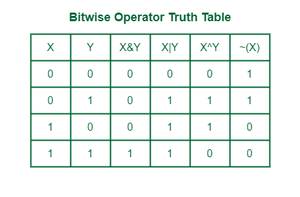Bitwise Operator Truth Table

## 1. Bitwise AND Operator (&)

The bitwise AND operator is denoted using a single ampersand symbol, i.e. &. The & operator takes two equal-length bit patterns as parameters. The two-bit integers are compared. If the bits in the compared positions of the bit patterns are 1, then the resulting bit is 1. If not, it is 0.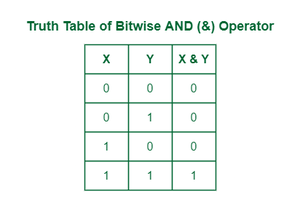Truth table of AND operator

Example:

Take two bit values X and Y, where X = 7= (111)2 and Y = 4 = (100)2 . Take Bitwise and of both X & y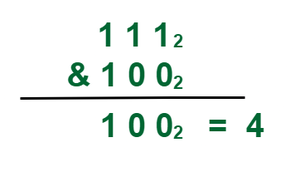Bitwise ANDof (7 & 4)

Implementation of AND operator:

## C++

 `#include ` `using` `namespace` `std; ` ` `  `int` `main() ` `{ ` ` `  `    ``int` `a = 7, b = 4; ` `    ``int` `result = a & b; ` `    ``cout << result << endl; ` ` `  `    ``return` `0; ` `}`

## Java

 `/*package whatever //do not write package name here */` ` `  `import` `java.io.*; ` ` `  `class` `GFG { ` `    ``public` `static` `void` `main (String[] args) { ` `        ``int` `a = ``7``, b = ``4``; ` `          ``int` `result = a & b; ` `          ``System.out.println(result); ` `    ``} ` `} ` ` `  `// This code is contributed by lokeshmvs21.`

## C#

 `using` `System; ` ` `  `public` `class` `GFG{ ` ` `  `    ``static` `public` `void` `Main (){ ` `      ``int` `a = 7, b = 4; ` `      ``int` `result = a & b; ` `      ``Console.WriteLine(result); ` `    ``} ` `} ` ` `  `// This code is contributed by akashish__`

## Python3

 `a ``=` `7` `b ``=` `4` `result ``=` `a & b ` `print``(result) ` `# This code is contributed by akashish__`

## Javascript

 `let a = 7, b = 4; ` `let result = a & b; ` `console.log(result); ` `// This code is contributed by akashish__`

Output

`4`

Time Complexity: O(1)
Auxiliary Space: O(1)

## 2 ​Bitwise OR Operator (|)

The | Operator takes two equivalent length bit designs as boundaries; if the two bits in the looked-at position are 0, the next bit is zero. If not, it is 1.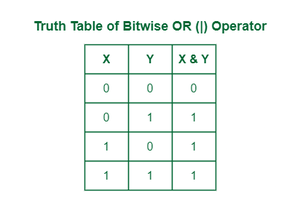Truth table of OR operator

Example:

Take two bit values X and Y, where X = 7= (111)2 and Y = 4 = (100)2 . Take Bitwise OR of both X, y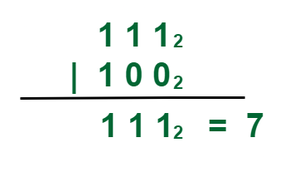Bitwise OR of (7 | 4)

Explanation: On the basis of truth table of bitwise OR operator we can conclude that the result of

1 | 1  = 1
1 | 0 = 1
0 | 1 = 1
0 | 0 = 0

We used the similar concept of bitwise operator that are show in the image.

Implementation of OR operator:

## C++

 `#include ` `using` `namespace` `std; ` ` `  `int` `main() ` `{ ` ` `  `    ``int` `a = 12, b = 25; ` `    ``int` `result = a | b; ` `    ``cout << result; ` ` `  `    ``return` `0; ` `}`

## Java

 `import` `java.io.*; ` ` `  `class` `GFG { ` `    ``public` `static` `void` `main(String[] args) ` `    ``{ ` `        ``int` `a = ``12``, b = ``25``; ` `        ``int` `result = a | b; ` `        ``System.out.println(result); ` `    ``} ` `}`

## Python3

 `a ``=` `12` `b ``=` `25` `result ``=` `a | b ` `print``(result) ` ` `  `# This code is contributed by garg28harsh.`

## C#

 `using` `System; ` ` `  `public` `class` `GFG{ ` ` `  `    ``static` `public` `void` `Main (){ ` `        ``int` `a = 12, b = 25; ` `        ``int` `result = a | b; ` `        ``Console.WriteLine(result); ` `    ``} ` `} ` `// This code is contributed by akashish__`

## Javascript

 `    ``let a = 12, b = 25; ` `    ``let result = a | b; ` `    ``document.write(result); ` `       `  `// This code is contributed by garg28harsh.`

Output

`29`

Time Complexity: O(1)
Auxiliary Space: O(1)

## 3. ​Bitwise XOR Operator (^)

The ^ operator (also known as the XOR operator) stands for Exclusive Or. Here, if bits in the compared position do not match their resulting bit is 1. i.e, The result of the bitwise XOR operator is 1 if the corresponding bits of two operands are opposite, otherwise 0.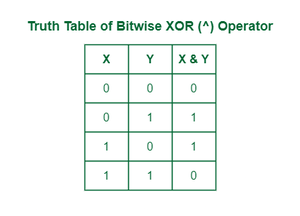Truth Table of Bitwise Operator XOR

Example:

Take two bit values X and Y, where X = 7= (111)2 and Y = 4 = (100)2 . Take Bitwise and of both X & y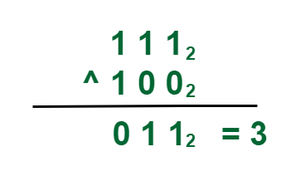Bitwise OR of (7 ^ 4)

Explanation: On the basis of truth table of bitwise XOR operator we can conclude that the result of

1 ^ 1  = 0
1 ^ 0 = 1
0 ^ 1 = 1
0 ^ 0 = 0

We used the similar concept of bitwise operator that are show in the image.

Implementation of XOR operator:

## C++

 `#include ` `using` `namespace` `std; ` ` `  `int` `main() ` `{ ` ` `  `    ``int` `a = 12, b = 25; ` `    ``cout << (a ^ b); ` `    ``return` `0; ` `}`

## Java

 `import` `java.io.*; ` ` `  `class` `GFG { ` `    ``public` `static` `void` `main(String[] args) ` `    ``{ ` `        ``int` `a = ``12``, b = ``25``; ` `        ``int` `result = a ^ b; ` `        ``System.out.println(result); ` `    ``} ` `} ` ` `  `// This code is contributed by garg28harsh.`

## Python3

 `a ``=` `12` `b ``=` `25` `result ``=` `a ^ b ` `print``(result) ` ` `  `# This code is contributed by garg28harsh.`

## C#

 `// C# Code ` ` `  `using` `System; ` ` `  `public` `class` `GFG { ` ` `  `    ``static` `public` `void` `Main() ` `    ``{ ` ` `  `        ``// Code ` `        ``int` `a = 12, b = 25; ` `        ``int` `result = a ^ b; ` `        ``Console.WriteLine(result); ` `    ``} ` `} ` ` `  `// This code is contributed by lokesh`

## Javascript

 `let a = 12; ` `let b = 25; ` `console.log((a ^ b)); ` `// This code is contributed by akashish__`

Output

`21`

Time Complexity: O(1)
Auxiliary Space: O(1)

## 4. ​Bitwise NOT Operator (!~)

All the above three bitwise operators are binary operators (i.e, requiring two operands in order to operate). Unlike other bitwise operators, this one requires only one operand to operate.

The bitwise Not Operator takes a single value and returns its one’s complement. The one’s complement of a binary number is obtained by toggling all bits in it, i.e, transforming the 0 bit to 1 and the 1 bit to 0.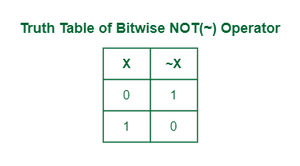Truth Table of Bitwise Operator NOT

Example:

Take two bit values X and Y, where X = 5= (101)2 . Take Bitwise NOT of X.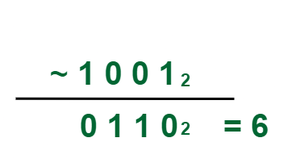Explanation: On the basis of truth table of bitwise NOT operator we can conclude that the result of

~1  = 0
~0 = 1

We used the similar concept of bitwise operator that are show in the image.

Implementation of NOT operator:

## C++

 `#include ` `using` `namespace` `std; ` ` `  `int` `main() ` `{ ` ` `  `    ``int` `a = 0; ` `    ``cout << ``"Value of a without using NOT operator: "` `<< a; ` `    ``cout << ``"\nInverting using NOT operator (with sign bit): "` `<< (~a); ` `    ``cout << ``"\nInverting using NOT operator (without sign bit): "` `<< (!a); ` ` `  `    ``return` `0; ` `}`

## Java

 `/*package whatever //do not write package name here */` ` `  `import` `java.io.*; ` ` `  `class` `GFG { ` `  ``public` `static` `void` `main(String[] args) ` `  ``{ ` `    ``int` `a = ``0``; ` `    ``System.out.println( ` `      ``"Value of a without using NOT operator: "` `+ a); ` `    ``System.out.println( ` `      ``"Inverting using NOT operator (with sign bit): "` `      ``+ (~a)); ` `    ``if` `(a != ``1``) ` `      ``System.out.println( ` `      ``"Inverting using NOT operator (without sign bit): 1"``); ` `    ``else` `      ``System.out.println( ` `      ``"Inverting using NOT operator (without sign bit): 0"``); ` `  ``} ` `} ` ` `  `// This code is contributed by lokesh.`

## Python3

 `a ``=` `0` `print``(``"Value of a without using NOT operator: "` `, a) ` `print``(``"Inverting using NOT operator (with sign bit): "` `, (~a)) ` `print``(``"Inverting using NOT operator (without sign bit): "` `, ``int``(``not``(a))) ` `#  This code is contributed by akashish__ `

## C#

 `using` `System; ` ` `  `public` `class` `GFG { ` ` `  `  ``static` `public` `void` `Main() ` `  ``{ ` ` `  `    ``int` `a = 0; ` `    ``Console.WriteLine( ` `      ``"Value of a without using NOT operator: "` `+ a); ` `    ``Console.WriteLine( ` `      ``"Inverting using NOT operator (with sign bit): "` `      ``+ (~a)); ` `    ``if` `(a != 1) ` `      ``Console.WriteLine( ` `      ``"Inverting using NOT operator (without sign bit): 1"``); ` `    ``else` `      ``Console.WriteLine( ` `      ``"Inverting using NOT operator (without sign bit): 0"``); ` `  ``} ` `} ` ` `  `// This code is contributed by akashish__`

## Javascript

 `let a =0; ` `document.write(``"Value of a without using NOT operator: "` `+ a); ` `document.write( ``"Inverting using NOT operator (with sign bit): "` `+ (~a)); ` `if``(!a) ` `document.write( ``"Inverting using NOT operator (without sign bit): 1"` `); ` `else` `document.write( ``"Inverting using NOT operator (without sign bit): 0"` `); `

Output

```Value of a without using NOT operator: 0
Inverting using NOT operator (with sign bit): -1
Inverting using NOT operator (without sign bit): 1```

Time Complexity: O(1)
Auxiliary Space: O(1)

## 5. Left-Shift (<<)

The left shift operator is denoted by the double left arrow key (<<). The general syntax for left shift is shift-expression << k. The left-shift operator causes the bits in shift expression to be shifted to the left by the number of positions specified by k. The bit positions that the shift operation has vacated are zero-filled.

Note: Every time we shift a number towards the left by 1 bit it multiply that number by 2.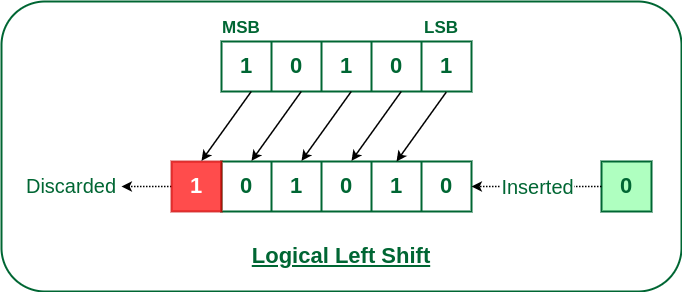Logical left Shift

Example:

Input: Left shift of 5 by 1.
Binary representation of 5 = 00101 and Left shift of 001012 by 1 (i.e, 00101 << 1)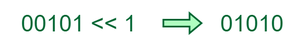Left shift of 5 by 1

Output: 10
Explanation: All bit of 5 will be shifted by 1 to left side and this result in 010102, Which is equivalent to 10

Input: Left shift of 5 by 2.
Binary representation of 5 = 00101 and Left shift of 001012 by 1 (i.e, 00101 << 2)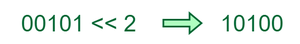Left shift of 5 by 2

Output: 20
Explanation: All bit of 5 will be shifted by 1 to left side and this result in 101002, Which is equivalent to 20

Input: Left shift of 5 by 3.
Binary representation of 5 = 00101 and Left shift of 001012 by 1 (i.e, 00101 << 3)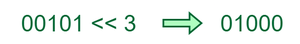Left shift of 5 by 3

Output: 40
Explanation: All bit of 5 will be shifted by 1 to left side and this result in 010002, Which is equivalent to 40

Implementation of Left shift operator:

## C++

 `#include ` `using` `namespace` `std; ` ` `  `int` `main() ` `{ ` `    ``unsigned ``int` `num1 = 1024; ` ` `  `    ``bitset<32> bt1(num1); ` `    ``cout << bt1 << endl; ` ` `  `    ``unsigned ``int` `num2 = num1 << 1; ` `    ``bitset<32> bt2(num2); ` `    ``cout << bt2 << endl; ` ` `  `    ``unsigned ``int` `num3 = num1 << 2; ` `    ``bitset<16> bitset13{ num3 }; ` `    ``cout << bitset13 << endl; ` `}`

## Java

 `/*package whatever //do not write package name here */` ` `  `import` `java.io.*; ` ` `  `class` `GFG { ` `  ``public` `static` `void` `main(String[] args) ` `  ``{ ` `    ``int` `num1 = ``1024``; ` ` `  `    ``String bt1 = Integer.toBinaryString(num1); ` `    ``bt1 = String.format(``"%32s"``, bt1).replace(``' '``, ``'0'``); ` `    ``System.out.println(bt1); ` ` `  `    ``int` `num2 = num1 << ``1``; ` `    ``String bt2 = Integer.toBinaryString(num2); ` `    ``bt2 = String.format(``"%32s"``, bt2).replace(``' '``, ``'0'``); ` `    ``System.out.println(bt2); ` ` `  `    ``int` `num3 = num1 << ``2``; ` `    ``String bitset13 = Integer.toBinaryString(num3); ` `    ``bitset13 = String.format(``"%16s"``, bitset13) ` `      ``.replace(``' '``, ``'0'``); ` `    ``System.out.println(bitset13); ` `  ``} ` `} ` ` `  `// This code is contributed by akashish__`

## Python3

 `# Python code for the above approach ` ` `  `num1 ``=` `1024` ` `  `bt1 ``=` `bin``(num1)[``2``:].zfill(``32``) ` `print``(bt1) ` ` `  `num2 ``=` `num1 << ``1` `bt2 ``=` `bin``(num2)[``2``:].zfill(``32``) ` `print``(bt2) ` ` `  `num3 ``=` `num1 << ``2` `bitset13 ``=` `bin``(num3)[``2``:].zfill(``16``) ` `print``(bitset13) ` ` `  `# This code is contributed by Prince Kumar`

## C#

 `using` `System; ` ` `  `class` `GFG { ` `  ``public` `static` `void` `Main(``string``[] args) ` `  ``{ ` `    ``int` `num1 = 1024; ` ` `  `    ``string` `bt1 = Convert.ToString(num1, 2); ` `    ``bt1 = bt1.PadLeft(32, ``'0'``); ` `    ``Console.WriteLine(bt1); ` ` `  `    ``int` `num2 = num1 << 1; ` `    ``string` `bt2 = Convert.ToString(num2, 2); ` `    ``bt2 = bt2.PadLeft(32, ``'0'``); ` `    ``Console.WriteLine(bt2); ` ` `  `    ``int` `num3 = num1 << 2; ` `    ``string` `bitset13 = Convert.ToString(num3, 2); ` `    ``bitset13 = bitset13.PadLeft(16, ``'0'``); ` `    ``Console.WriteLine(bitset13); ` `  ``} ` `} ` ` `  `// This code is contributed by akashish__`

## Javascript

 `// JavaScript code for the above approach ` ` `  `let num1 = 1024; ` ` `  `let bt1 = num1.toString(2).padStart(32, ``'0'``); ` `console.log(bt1); ` ` `  `let num2 = num1 << 1; ` `let bt2 = num2.toString(2).padStart(32, ``'0'``); ` `console.log(bt2); ` ` `  `let num3 = num1 << 2; ` `let bitset13 = num3.toString(2).padStart(16, ``'0'``); ` `console.log(bitset13);`

Output

```00000000000000000000010000000000
00000000000000000000100000000000
0001000000000000```

Time Complexity: O(1)
Auxiliary Space: O(1)

## 6. Right-Shift (>>)

The right shift operator is denoted by the double right arrow key (>>). The general syntax for the right shift is “shift-expression >> k”. The right-shift operator causes the bits in shift expression to be shifted to the right by the number of positions specified by k. For unsigned numbers, the bit positions that the shift operation has vacated are zero-filled. For signed numbers, the sign bit is used to fill the vacated bit positions. In other words, if the number is positive, 0 is used, and if the number is negative, 1 is used.

Note: Every time we shift a number towards the right by 1 bit it divides that number by 2.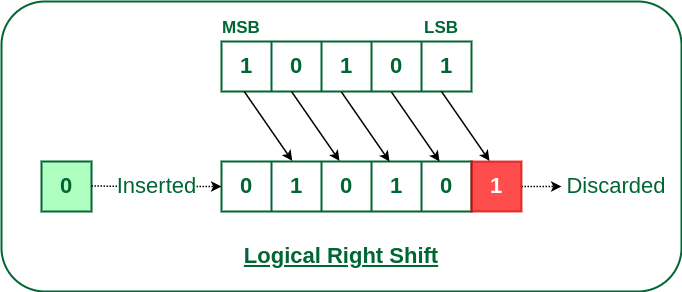Logical Right Shift

Example:

Input: Left shift of 5 by 1.
Binary representation of 5 = 00101 and Left shift of 001012 by 1 (i.e, 00101 << 1)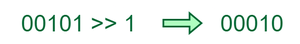Right shift of 5 by 1

Output: 10
Explanation: All bit of 5 will be shifted by 1 to left side and this result in 010102, Which is equivalent to 10

Input: Left shift of 5 by 2.
Binary representation of 5 = 00101 and Left shift of 001012 by 1 (i.e, 00101 << 2)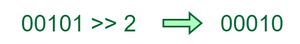Right shift of 5 by 2

Output: 20
Explanation: All bit of 5 will be shifted by 1 to left side and this result in 101002, Which is equivalent to 20

Input: Left shift of 5 by 3.
Binary representation of 5 = 00101 and Left shift of 001012 by 1 (i.e, 00101 << 3)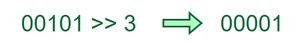Right shift of 5 by 3

Output: 40
Explanation: All bit of 5 will be shifted by 1 to left side and this result in 010002, Which is equivalent to 40

Implementation of Right shift operator:

## C++

 `#include ` `#include ` ` `  `using` `namespace` `std; ` ` `  `int` `main() ` `{ ` `    ``unsigned ``int` `num1 = 1024; ` ` `  `    ``bitset<32> bt1(num1); ` `    ``cout << bt1 << endl; ` ` `  `    ``unsigned ``int` `num2 = num1 >> 1; ` `    ``bitset<32> bt2(num2); ` `    ``cout << bt2 << endl; ` ` `  `    ``unsigned ``int` `num3 = num1 >> 2; ` `    ``bitset<16> bitset13{ num3 }; ` `    ``cout << bitset13 << endl; ` `}`

## Javascript

 `// JavaScript code for the above approach ` ` `  `let num1 = 1024; ` ` `  `let bt1 = num1.toString(2).padStart(32, ``'0'``); ` `console.log(bt1); ` ` `  `let num2 = num1 >> 1; ` `let bt2 = num2.toString(2).padStart(32, ``'0'``); ` `console.log(bt2); ` ` `  `let num3 = num1 >> 2; ` `let bitset13 = num3.toString(2).padStart(16, ``'0'``); ` `console.log(bitset13); ` `// akashish__`

Output

```00000000000000000000010000000000
00000000000000000000001000000000
0000000100000000```

Time Complexity: O(1)
Auxiliary Space: O(1)

## Application of BIT Operators

• Bit operations are used for the optimization of embedded systems.
• The Exclusive-or operator can be used to confirm the integrity of a file, making sure it has not been corrupted, especially after it has been in transit.
• Bitwise operations are used in Data encryption and compression.
• Bits are used in the area of networking, framing the packets of numerous bits which are sent to another system generally through any type of serial interface.
• Digital Image Processors use bitwise operations to enhance image pixels and to extract different sections of a microscopic image.

## Important Practice Problems on Bitwise Algorithm:

### 1. How to Set a bit in the number?

If we want to set a bit at nth position in the number ‘num’, it can be done using the ‘OR’ operator( | ).

• First, we left shift 1 to n position via (1<<n)
• Then, use the “OR” operator to set the bit at that position. “OR” operator is used because it will set the bit even if the bit is unset previously in the binary representation of the number ‘num’.

Note: If the bit would be already set then it would remain unchanged.

Below is the implementation:

## C++

 `#include ` `using` `namespace` `std; ` `// num is the number and pos is the position ` `// at which we want to set the bit. ` `void` `set(``int``& num, ``int` `pos) ` `{ ` `    ``// First step is shift '1', second ` `    ``// step is bitwise OR ` `    ``num |= (1 << pos); ` `} ` `int` `main() ` `{ ` `    ``int` `num = 4, pos = 1; ` `    ``set(num, pos); ` `    ``cout << (``int``)(num) << endl; ` `    ``return` `0; ` `}`

## Java

 `/*package whatever //do not write package name here */` ` `  `import` `java.io.*; ` ` `  `class` `GFG { ` `    ``public` `static` `void` `main(String[] args) ` `    ``{ ` `        ``int` `num = ``4``, pos = ``1``; ` `        ``num = set(num, pos); ` `        ``System.out.println(num); ` `    ``} ` `    ``public` `static` `int` `set(``int` `num, ``int` `pos) ` `    ``{ ` `        ``// First step is shift '1', second ` `        ``// step is bitwise OR ` `        ``num |= (``1` `<< pos); ` `        ``return` `num; ` `    ``} ` `} ` ` `  `// This code is contributed by geeky01adash.`

## Python3

 `# num = number, pos = position at which we want to set the bit ` `def` `set``(num, pos): ` `    ``# First step = Shift '1' ` `    ``# Second step = Bitwise OR ` `    ``num |``=` `(``1` `<< pos) ` `    ``print``(num) ` ` `  ` `  `num, pos ``=` `4``, ``1` ` `  `set``(num, pos) ` ` `  `# This code is contributed by sarajadhav12052009 `

## C#

 `using` `System; ` ` `  `public` `class` `GFG { ` ` `  `    ``static` `public` `void` `Main() ` `    ``{ ` `        ``int` `num = 4, pos = 1; ` `        ``set``(num, pos); ` `    ``} ` ` `  `    ``// num = number, pos = position at which we want to set ` `    ``// the bit ` `    ``static` `public` `void` `set``(``int` `num, ``int` `pos) ` `    ``{ ` `        ``// First Step: Shift '1' ` `        ``// Second Step: Bitwise OR ` `        ``num |= (1 << pos); ` `        ``Console.WriteLine(num); ` `    ``} ` `} ` ` `  `// This code is contributed by sarajadhav12052009`

## Javascript

 ``

Output

`6`

Time Complexity: O(1)
Auxiliary Space: O(1)

### 2. How to unset/clear a bit at n’th position in the number

Suppose we want to unset a bit at nth position in number ‘num’ then we have to do this with the help of “AND” (&) operator.

• First, we left shift ‘1’ to n position via (1<<n) then we use bitwise NOT operator ‘~’ to unset this shifted ‘1’.
• Now after clearing this left shifted ‘1’ i.e making it to ‘0’ we will ‘AND'(&) with the number ‘num’ that will unset bit at nth position.

Below is the implementation:

## C++

 `#include ` `using` `namespace` `std; ` `// First step is to get a number that  has all 1's except ` `// the given position. ` `void` `unset(``int``& num, ``int` `pos) ` `{ ` `    ``// Second step is to bitwise and this  number with given ` `    ``// number ` `    ``num &= (~(1 << pos)); ` `} ` `int` `main() ` `{ ` `    ``int` `num = 7; ` `    ``int` `pos = 1; ` `    ``unset(num, pos); ` `    ``cout << num << endl; ` `    ``return` `0; ` `}`

## Java

 `/*package whatever //do not write package name here */` ` `  `import` `java.io.*; ` ` `  `class` `GFG { ` `    ``public` `static` `void` `main(String[] args) ` `    ``{ ` `        ``int` `num = ``7``, pos = ``1``; ` `        ``num = unset(num, pos); ` `        ``System.out.println(num); ` `    ``} ` `    ``public` `static` `int` `unset(``int` `num, ``int` `pos) ` `    ``{ ` `        ``// Second step is to bitwise and this  number with ` `        ``// given number ` `        ``num = num & (~(``1` `<< pos)); ` `        ``return` `num; ` `    ``} ` `}`

## Python3

 `# First Step: Getting which have all '1's except the ` `# given position ` ` `  ` `  `def` `unset(num, pos): ` `    ``# Second Step: Bitwise AND this number with the given number ` `    ``num &``=` `(~(``1` `<< pos)) ` `    ``print``(num) ` ` `  ` `  `num, pos ``=` `7``, ``1` ` `  `unset(num, pos) `

## C#

 `using` `System; ` ` `  `public` `class` `GFG { ` ` `  `    ``static` `public` `void` `Main() ` `    ``{ ` `        ``// First Step: Getting a number which have all '1's ` `        ``// except the given position ` `        ``int` `num = 7, pos = 1; ` `        ``unset(num, pos); ` `    ``} ` `    ``static` `public` `void` `unset(``int` `num, ``int` `pos) ` `    ``{ ` `        ``// Second Step: Bitwise AND this number with the ` `        ``// given number ` `        ``num &= (~(1 << pos)); ` `        ``Console.WriteLine(num); ` `    ``} ` `}`

## Javascript

 `// First step is to get a number that  has all 1's except ` `// the given position. ` `function` `unset(num, pos) ` `{ ` `    ``// Second step is to bitwise and this  number with given ` `    ``// number ` `    ``return` `num &= (~(1 << pos)); ` `} ` ` `  `let num = 7; ` `let pos = 1; ` `console.log(unset(num, pos)); ` ` `  `// contributed by akashish__`

Output

`5`

Time Complexity: O(1)
Auxiliary Space: O(1)

### 3. Toggling a bit at nth position

Toggling means to turn bit ‘on'(1) if it was ‘off'(0) and to turn ‘off'(0) if it was ‘on'(1) previously. We will be using the ‘XOR’ operator here which is this ‘^’. The reason behind the ‘XOR’ operator is because of its properties.

• Properties of ‘XOR’ operator.
• 1^1 = 0
• 0^0 = 0
• 1^0 = 1
• 0^1 = 1
• If two bits are different then the ‘XOR’ operator returns a set bit(1) else it returns an unset bit(0).

Below is the implementation:

## C++

 `#include ` `using` `namespace` `std; ` `// First step is to shift 1,Second step is to XOR with given ` `// number ` `void` `toggle(``int``& num, ``int` `pos) { num ^= (1 << pos); } ` `int` `main() ` `{ ` `    ``int` `num = 4; ` `    ``int` `pos = 1; ` `    ``toggle(num, pos); ` `    ``cout << num << endl; ` `    ``return` `0; ` `}`

## Java

 `/*package whatever //do not write package name here */` ` `  `import` `java.io.*; ` ` `  `class` `GFG { ` `    ``public` `static` `void` `main(String[] args) ` `    ``{ ` `        ``int` `num = ``4``, pos = ``1``; ` `        ``num = toggle(num, pos); ` `        ``System.out.println(num); ` `    ``} ` `    ``public` `static` `int` `toggle(``int` `num, ``int` `pos) ` `    ``{ ` `        ``// First step is to shift 1,Second step is to XOR ` `        ``// with given number ` `        ``num ^= (``1` `<< pos); ` `        ``return` `num; ` `    ``} ` `} ` ` `  `// This code is contributed by geeky01adash.`

## Python3

 `def` `toggle(num, pos): ` `    ``# First Step: Shifts '1' ` `    ``# Second Step: XOR num ` `    ``num ^``=` `(``1` `<< pos) ` `    ``print``(num) ` ` `  ` `  `num, pos ``=` `4``, ``1` ` `  `toggle(num, pos) ` ` `  `# This code is contributed by sarajadhav12052009 `

## C#

 `using` `System; ` ` `  `public` `class` `GFG { ` ` `  `    ``static` `public` `void` `Main() ` `    ``{ ` `        ``int` `num = 4, pos = 1; ` `        ``toggle(num, pos); ` `    ``} ` `    ``static` `public` `void` `toggle(``int` `num, ``int` `pos) ` `    ``{ ` `        ``// First Step: Shift '1' ` `        ``// Second Step: XOR num ` `        ``num ^= (1 << pos); ` `        ``Console.WriteLine(num); ` `    ``} ` `} ` ` `  `// This code is contributed by sarajadhav12052009`

## Javascript

 `// First step is to shift 1,Second step is to XOR with given ` `// number ` `function` `toggle(num, pos){ ` `    ``// First Step: Shifts '1' ` `    ``// Second Step: XOR num ` `    ``num ^= (1 << pos) ` `    ``console.log(num) ` `} ` ` `  `let num = 4; ` `let pos = 1; ` `toggle(num, pos); ` `// contributed by akashish__`

Output

`6`

Time Complexity: O(1)
Auxiliary Space: O(1)

### 4. Checking if the bit at nth position is Set or Unset

We used the left shift (<<) operation on 1 to shift the bits to nth position and then use the & operation with number given number, and check if it is not-equals to 0.

Below is the implementation:

## C++

 `#include ` `using` `namespace` `std; ` ` `  `bool` `at_position(``int` `num, ``int` `pos) ` `{ ` `    ``bool` `bit = num & (1 << pos); ` `    ``return` `bit; ` `} ` ` `  `int` `main() ` `{ ` `    ``int` `num = 5; ` `    ``int` `pos = 2; ` `    ``bool` `bit = at_position(num, pos); ` `    ``cout << bit << endl; ` `    ``return` `0; ` `}`

## Java

 `/*package whatever //do not write package name here */` ` `  `import` `java.io.*; ` ` `  `class` `GFG { ` `    ``public` `static` `void` `main(String[] args) ` `    ``{ ` `        ``int` `num = ``5``; ` `        ``int` `pos = ``0``; ` `        ``int` `bit = at_position(num, pos); ` `        ``System.out.println(bit); ` `    ``} ` `    ``public` `static` `int` `at_position(``int` `num, ``int` `pos) ` `    ``{ ` `        ``int` `bit = num & (``1` `<< pos); ` `        ``return` `bit; ` `    ``} ` `}`

## Python3

 `# code ` `def` `at_position(num, pos): ` `    ``bit ``=` `num & (``1` `<< pos) ` `    ``return` `bit ` ` `  ` `  `num ``=` `5` `pos ``=` `0` `bit ``=` `at_position(num, pos) ` `print``(bit) `

## C#

 `using` `System; ` ` `  `public` `class` `GFG { ` ` `  `  ``public` `static` `bool` `at_position(``int` `num, ``int` `pos) ` `  ``{ ` `    ``int` `bit = num & (1 << pos); ` `    ``if` `(bit == 0) ` `      ``return` `false``; ` `    ``return` `true``; ` `  ``} ` ` `  `  ``static` `public` `void` `Main() ` `  ``{ ` `    ``int` `num = 5; ` `    ``int` `pos = 2; ` `    ``bool` `bit = at_position(num, pos); ` `    ``Console.WriteLine(bit); ` `  ``} ` `} ` ` `  `// This code is contributed by akashish__`

## Javascript

 ``

Output

`1`

Time Complexity: O(1)
Auxiliary Space: O(1)

### 5. Multiply a number by 2 using the left shift operator

Below is the implementation:

## C++

 `#include ` `using` `namespace` `std; ` `int` `main() ` `{ ` `    ``int` `num = 12; ` `    ``int` `ans = num << 1; ` `    ``cout << ans << endl; ` `    ``return` `0; ` `}`

## Java

 `/*package whatever //do not write package name here */` ` `  `import` `java.io.*; ` ` `  `class` `GFG { ` `    ``public` `static` `void` `main(String[] args) ` `    ``{ ` `        ``int` `num = ``12``; ` `        ``int` `ans = num << ``1``; ` `        ``System.out.println(ans); ` `    ``} ` `} ` ` `  `// This code is contributed by geeky01adash.`

## C#

 `using` `System; ` ` `  `public` `class` `GFG { ` ` `  `    ``static` `public` `void` `Main() ` `    ``{ ` `        ``int` `num = 12; ` `        ``Console.WriteLine(num << 1); ` `    ``} ` `} ` ` `  `// This code is contributed by sarajadhav12052009`

## Python3

 `# Python program for the above approach ` ` `  `num ``=` `12` `ans ``=` `num << ``1` `print``(ans) ` ` `  `# This code is contributed by Shubham Singh `

## Javascript

 ``

Output

`24`

Time Complexity: O(1)
Auxiliary Space: O(1)

### 6. Divide a number 2 using the right shift operator

Below is the implementation:

## C++

 `#include ` `using` `namespace` `std; ` `int` `main() ` `{ ` `    ``int` `num = 12; ` `    ``int` `ans = num >> 1; ` `    ``cout << ans << endl; ` `    ``return` `0; ` `}`

## Java

 `/*package whatever //do not write package name here */` ` `  `import` `java.io.*; ` ` `  `class` `GFG { ` `    ``public` `static` `void` `main(String[] args) ` `    ``{ ` `        ``int` `num = ``12``; ` `        ``int` `ans = num >> ``1``; ` `        ``System.out.println(ans); ` `    ``} ` `} ` ` `  `// This code is contributed by geeky01adash.`

## C#

 `using` `System; ` ` `  `public` `class` `GFG { ` ` `  `    ``static` `public` `void` `Main() ` `    ``{ ` `        ``int` `num = 12; ` `        ``Console.WriteLine(num >> 1); ` `    ``} ` `} ` ` `  `// This code is contributed by sarajadhav12052009`

## Python3

 `# Python program for the above approach ` ` `  `num ``=` `12` `ans ``=` `num >> ``1` `print``(ans) ` ` `  `# This code is contributed by Shubham Singh `

## Javascript

 ``

Time Complexity: O(1)
Auxiliary Space: O(1)

### 7. Compute XOR from 1 to n (direct method)

The  problem can be solved based on the following observations:

Say x = n % 4. The XOR value depends on the value if x.

If, x = 0, then the answer is n.
x = 1, then answer is 1.
x = 2, then answer is n+1.
x = 3, then answer is 0.

Below is the implementation of the above approach.

## C++

 `// Direct XOR of all numbers from 1 to n ` `int` `computeXOR(``int` `n) ` `{ ` `    ``if` `(n % 4 == 0) ` `        ``return` `n; ` `    ``if` `(n % 4 == 1) ` `        ``return` `1; ` `    ``if` `(n % 4 == 2) ` `        ``return` `n + 1; ` `    ``else` `        ``return` `0; ` `}`

## Java

 `/*package whatever //do not write package name here */` `import` `java.io.*; ` ` `  `class` `GFG { ` ` `  `    ``// Direct XOR of all numbers from 1 to n ` `    ``public` `static` `int` `computeXOR(``int` `n) ` `    ``{ ` `        ``if` `(n % ``4` `== ``0``) ` `            ``return` `n; ` `        ``if` `(n % ``4` `== ``1``) ` `            ``return` `1``; ` `        ``if` `(n % ``4` `== ``2``) ` `            ``return` `n + ``1``; ` `        ``else` `            ``return` `0``; ` `    ``} ` ` `  `    ``public` `static` `void` `main(String[] args) {} ` `} ` ` `  `// This code is contributed by akashish__`

## Python

 `# num = number, pos = position at which we want to set the bit ` `def` `set``(num, pos): ` ` `  `    ``# First step = Shift '1' ` `    ``# Second step = Bitwise OR ` `num |``=` `(``1` `<< pos) ` `print``(num) ` ` `  `num, pos ``=` `4``, ``1` ` `  `set``(num, pos) ` ` `  `# This code is contributed by sarajadhav12052009 `

## C#

 `using` `System; ` `public` `class` `GFG { ` ` `  `    ``// Direct XOR of all numbers from 1 to n ` `    ``public` `static` `int` `computeXOR(``int` `n) ` `    ``{ ` ` `  `        ``if` `(n % 4 == 0) ` ` `  `            ``return` `n; ` ` `  `        ``if` `(n % 4 == 1) ` ` `  `            ``return` `1; ` ` `  `        ``if` `(n % 4 == 2) ` ` `  `            ``return` `n + 1; ` ` `  `        ``else` ` `  `            ``return` `0; ` `    ``} ` `    ``public` `static` `void` `Main() {} ` `} ` ` `  `// This code is contributed by akashish__`

## Javascript

 ``

Time Complexity: O(1)
Auxiliary Space: O(1)

### 8. How to know if a number is a power of 2?

This can be solved based on the following fact:

If a number N is a power of 2, then the bitwise AND of N and N-1 will be 0. But this will not work if N is 0. So just check these two conditions, if any of these two conditions is true.

Below is the implementation of the above approach.

## C++

 `// Function to check if x is power of 2 ` `bool` `isPowerOfTwo(``int` `x) ` `{ ` `    ``// First x in the below expression is ` `    ``// for the case when x is 0 ` `    ``return` `x && (!(x & (x - 1))); ` `}`

## Java

 `// Function to check if x is power of 2 ` `public` `static` `boolean` `isPowerOfTwo(``int` `x) ` `{ ` `    ``// First x in the below expression is ` `    ``// for the case when x is 0 ` `    ``return` `x != ``0` `&& ((x & (x - ``1``)) == ``0``); ` `}`

## Python

 `# Function to check if x is power of 2 ` `def` `isPowerOfTwo(x): ` ` `  ` `  `    ``# First x in the below expression is ` `    ``# for the case when x is 0 ` `return` `x ``and` `(``not``(x & (x ``-` `1``))) ` ` `  `# This code is contributed by akashish__ `

## C#

 `using` `System; ` ` `  `public` `class` `GFG { ` ` `  `    ``// Function to check if x is power of 2 ` `    ``static` `public` `bool` `isPowerOfTwo(``int` `x) ` `    ``{ ` `        ``// First x in the below expression is ` `        ``// for the case when x is 0 ` `        ``return` `(x != 0) && ((x & (x - 1)) == 0); ` `    ``} ` ` `  `    ``static` `public` `void` `Main() {} ` `} ` ` `  `// This code is contributed by akashish__`

## Javascript

 `// Function to check if x is power of 2 ` `function` `isPowerOfTwo(x) ` `{ ` `    ``// First x in the below expression is ` `    ``// for the case when x is 0 ` `    ``return` `x && (!(x & (x - 1))); ` `} ` `// contributed by akashish__`

Time Complexity: O(1)
Auxiliary Space: O(1)

### 9. Count Set bits in an integer

Counting set bits means, counting total number of 1’s in the binary representation of an integer. For this problem we go through all the bits of given number and check whether it is set or not by performing AND operation (with 1).

Below is the implementation:

## C++

 `// Function to calculate the number of set bits. ` `int` `countBits(``int` `n) ` `{ ` `    ``// Initialising a variable count to 0. ` `    ``int` `count = 0; ` `    ``while` `(n) { ` `        ``// If the last bit is 1, count will be incremented ` `        ``// by 1 in this step. ` `        ``count += n & 1; ` ` `  `        ``// Using the right shift operator. ` `        ``// The bits will be shifted one position to the ` `        ``// right. ` `        ``n >>= 1; ` `    ``} ` `    ``return` `count; ` `}`

## Java

 `// Function to calculate the number of set bits. ` `public` `static` `int` `countBits(``int` `n) ` `{ ` `    ``// Initialising a variable count to 0. ` `    ``int` `count = ``0``; ` `    ``while` `(n > ``0``) { ` `        ``// If the last bit is 1, count will be incremented ` `        ``// by 1 in this step. ` `        ``count += n & ``1``; ` ` `  `        ``// Using the right shift operator. ` `        ``// The bits will be shifted one position to the ` `        ``// right. ` `        ``n >>= ``1``; ` `    ``} ` `    ``return` `count; ` `}`

## Python3

 `def` `countBits(n): ` `    ``# Initializing a variable count to 0 ` `    ``count ``=` `0` `    ``while` `n: ` `        ``# If the last bit is 1, count will be incremented by 1 in this step. ` `        ``count ``+``=` `n & ``1` `        ``# Using the right shift operator. The bits will be shifted one position to the right. ` `        ``n >>``=` `1` `    ``return` `count `

## C#

 `using` `System; ` ` `  `public` `class` `GFG { ` ` `  `  ``// Function to calculate the number of set bits. ` `  ``public` `static` `int` `countBits(``int` `n) ` `  ``{ ` ` `  `    ``// Initialising a variable count to 0. ` `    ``int` `count = 0; ` `    ``while` `(n > 0) ` `    ``{ ` ` `  `      ``// If the last bit is 1, count will be ` `      ``// incremented by 1 in this step. ` `      ``count += n & 1; ` ` `  `      ``// Using the right shift operator. ` `      ``// The bits will be shifted one position to the ` `      ``// right. ` `      ``n >>= 1; ` `    ``} ` `    ``return` `count; ` `  ``} ` ` `  `  ``static` `public` `void` `Main() {} ` `} ` ` `  `// This code is contributed by akashish__`

## Javascript

 `// Function to calculate the number of set bits. ` `function` `countBits(n) ` `{ ` `    ``// Initialising a variable count to 0. ` `    ``let count = 0; ` `    ``while` `(n) { ` `        ``// If the last bit is 1, count will be incremented ` `        ``// by 1 in this step. ` `        ``count += n & 1; ` ` `  `        ``// Using the right shift operator. ` `        ``// The bits will be shifted one position to the ` `        ``// right. ` `        ``n >>= 1; ` `    ``} ` `    ``return` `count; ` `}`

Time Complexity: O(log(n))
Auxiliary Space: O(1)

### 10. Position of rightmost set bit

The idea is to unset the rightmost bit of number n and XOR the result with n. Then the rightmost set bit in n will be the position of the only set bit in the result. Note that if n is odd, we can directly return 1 as the first bit is always set for odd numbers.

Example:
The number 20 in binary is 00010100, and the position of the rightmost set bit is 3.

00010100    &               (n = 20)
00010011                     (n-1 = 19)
——————-
00010000    ^                (XOR result number with n)
00010100
——————-
00000100 ——->  rightmost set bit will tell us the position

Below is the implementation:

## C++

 `// Returns the position of the rightmost set bit of `n` ` `int` `positionOfRightmostSetBit(``int` `n) ` `{ ` `    ``// if the number is odd, return 1 ` `    ``if` `(n & 1) { ` `        ``return` `1; ` `    ``} ` ` `  `    ``// unset rightmost bit and xor with the number itself ` `    ``n = n ^ (n & (n - 1)); ` ` `  `    ``// find the position of the only set bit in the result; ` `    ``// we can directly return `log2(n) + 1` from the ` `    ``// function ` `    ``int` `pos = 0; ` `    ``while` `(n) { ` `        ``n = n >> 1; ` `        ``pos++; ` `    ``} ` `    ``return` `pos; ` `} `

## Java

 `// Returns the position of the rightmost set bit of `n` ` `public` `static` `int` `positionOfRightmostSetBit(``int` `n) ` `{ ` `    ``// if the number is odd, return 1 ` `    ``if` `((n & ``1``) != ``0``) { ` `        ``return` `1``; ` `    ``} ` ` `  `    ``// unset rightmost bit and xor with the number itself ` `    ``n = n ^ (n & (n - ``1``)); ` ` `  `    ``// find the position of the only set bit in the result; ` `    ``// we can directly return `log2(n) + 1` from the ` `    ``// function ` `    ``int` `pos = ``0``; ` `    ``while` `(n != ``0``) { ` `        ``n = n >> ``1``; ` `        ``pos++; ` `    ``} ` ` `  `    ``return` `pos; ` `}`

## Python

 `# Returns the position of the rightmost set bit of `n` ` ` `  ` `  `def` `positionOfRightmostSetBit(n): ` `  ``# if the number is odd, return 1 ` `    ``if` `n & ``1``: ` `        ``return` `1` ` `  `    ``# unset rightmost bit and xor with the number itself ` `    ``n ``=` `n ^ (n & (n ``-` `1``)) ` ` `  `    ``# find the position of the only set bit in the result; ` `    ``# we can directly return `log2(n) + 1` from the function ` `    ``pos ``=` `0` `    ``while` `n: ` `        ``n ``=` `n >> ``1` `        ``pos ``=` `pos ``+` `1` ` `  `    ``return` `pos ` `  `

## C#

 `// Returns the position of the rightmost set bit of `n` ` `public` `static` `int` `positionOfRightmostSetBit(``int` `n) ` `{ ` `    ``// if the number is odd, return 1 ` `    ``if` `((n & 1) != 0) { ` `        ``return` `1; ` `    ``} ` ` `  `    ``// unset rightmost bit and xor with the number itself ` `    ``n = n ^ (n & (n - 1)); ` ` `  `    ``// find the position of the only set bit in the result; ` `    ``// we can directly return `log2(n) + 1` from the ` `    ``// function ` `    ``int` `pos = 0; ` `    ``while` `(n != 0) { ` `        ``n = n >> 1; ` `        ``pos++; ` `    ``} ` ` `  `    ``return` `pos; ` `}`

## Javascript

 `// Returns the position of the rightmost set bit of `n` ` `function` `positionOfRightmostSetBit( n) ` `{ ` `    ``// if the number is odd, return 1 ` `    ``if` `(n & 1) { ` `        ``return` `1; ` `    ``} ` ` `  `    ``// unset rightmost bit and xor with the number itself ` `    ``n = n ^ (n & (n - 1)); ` ` `  `    ``// find the position of the only set bit in the result; ` `    ``// we can directly return `log2(n) + 1` from the ` `    ``// function ` `    ``let pos = 0; ` `    ``while` `(n) { ` `        ``n = n >> 1; ` `        ``pos++; ` `    ``} ` `    ``return` `pos; ` `} `

Time Complexity: O(log(n))
Auxiliary Space: O(1)

## More Practice Problems on Bitwise Algorithms

Related article:

My Personal Notes arrow_drop_up